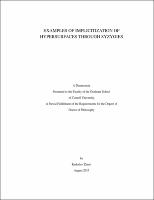## Examples Of Implicitization Of Hypersurfaces Through Syzygies##### Abstract
Let X be a smooth projective toric variety of dimension n [-] 1 and let [phi] : X [-][RIGHTWARDS ARROW] Pn be a generically finite rational map. The closed image Y can be defined by a single equation P(x), called the implicit equation. The implicitization problem asks for techniques for finding the implicit equation. This is an old problem in algebraic geometry, and can be solved effectively through elimination using Gr¨ bner bases. However, this solution represents a black box in relao tion to the geometry on the base locus and the closed image, and is unfeasible even for reasonably small examples. In this thesis, we use ideas from the two most popular non-Gr¨ bner basis approaches, o the method of the approximation complex and the method of moving surfaces, to construct a family of matrices N, one for each element in Pic(X), capturing determinantal representations for P(x). An algorithm for this calculation is described and implemented in the Macaulay2 system. Example calculations in previously intractable situations are presented.
2015-08-17
##### Committee Chair
Stillman,Michael Eugene
Swartz,Edward B.
Knutson,Allen
Mathematics
##### Degree Name
Ph. D., Mathematics
##### Degree Level
Doctor of Philosophy
##### Types
dissertation or thesis